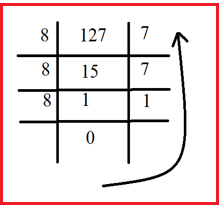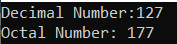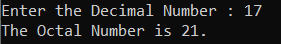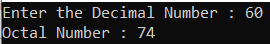# Decimal to Octal Conversion in C#

## Decimal to Octal Conversion in C# with Examples

In this article, I am going to discuss Decimal Number to Octal Number Conversion in C# with Examples. Please read our previous article, where we discussed Binary to Octal Number Conversion in C#. At the end of this article, you will understand how to convert a decimal number to an octal number in C#.

##### Decimal Number System in Computer:

Decimal is a term that describes the base-10 number system. The decimal number system consists of ten single-digit numbers: 0, 1, 2, 3, 4, 5, 6, 7, 8, and 9. Example: (35)10

##### Octal Number System in Computer:

The octal numeral system or oct describes the base-8 number system and uses the digits 0 to 7. Octal numerals can be made from binary numerals by grouping consecutive binary digits into groups of three (starting from the right). Example: (5303)8

##### Decimal to octal conversion example:

Decimal number ((127)10

1. Divide 127 by 8. 127 ÷ 8= 15(Quotient) and (7) Remainder
2. Divide 15 by 8 again. 15 ÷ 8 = 1(Quotient) and (7) Remainder
3. Divide 1 by 8, we get. 1 ÷ 8 = 0(Quotient) and (1) Remainder

The quotient is 0 now, no more division can be possible. So, by taking the remainders in reverse order, we get the octal number. Hence, (127)10 = (177)8. For better understanding, please have a look at the below image.##### Logic to Convert Decimal to Octal in C#

To find the conversion of Decimal to Octal number simply need to follow the below steps:

1. First, we divide the decimal numbers by 8.
2. Then we get quotient and remainder.
3. If the remainder is greater than 0 then again, we repeat step 1 and step 2.
4. Then we store the number from bottom to top and we get the octal number
##### Example: Decimal to Octal Conversion in C#

The following C# Program will convert a decimal number to its equivalent octal number.

```using System;
class Demo
{
public static void Main()
{
int decValue, counter, i = 1, j;
int[] octalValue = new int;
decValue = 127;
counter = decValue;
Console.WriteLine("Decimal Number:{0}", decValue);
while (counter != 0)
{
octalValue[i++] = counter % 8;
counter /= 8;
}

Console.Write("Octal Number: ");
for (j = i - 1; j > 0; j--)
Console.Write(octalValue[j]);
}
}
```
###### Output:##### Example: Taking input from the user

In the following C# program, we will take the decimal number from the user and then convert the decimal number to its equivalent octal number and print it on the console.

```using System;
public class DecimaltoOctal
{
public static void Main()
{
int i, j, Octal = 0, dn;
Console.Write("Enter the Decimal Number : ");
dn = DecimalNumber;
i = 1;

for (j = DecimalNumber; j > 0; j = j / 8)
{
Octal = Octal + (j % 8) * i;
i *= 10;
DecimalNumber /= 8;
}

Console.WriteLine("The Octal Number is {1}.\n\n", dn, Octal);
}
}
```
###### Output:##### C# Code (Using function)

In the below example, we have created one method to convert the decimal number to an octal number.

```using System;
class Decimaltooctal
{
static void DecToOctal(int n)
{
int[] octalNum = new int;
int i = 0;
while (n != 0)
{
octalNum[i] = n % 8;
n /= 8;
i++;
}
Console.Write("Octal Number : ");
for (int j = i - 1; j >= 0; j--)
Console.Write(octalNum[j]);
}
public static void Main()
{
Console.Write("Enter the Decimal Number : ");